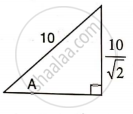# Find Angle 'A' If : - Mathematics

Sum

Find angle 'A' if :#### Solution

From the figure we have

sin A = ((10)/sqrt(2))/(10)

sin A =(1)/(sqrt(2)

sin A = sin 45°

A = 45°

Concept: Solution of Right Triangles
Is there an error in this question or solution?

#### APPEARS IN

Selina Concise Mathematics Class 9 ICSE
Chapter 24 Solution of Right Triangles [Simple 2-D Problems Involving One Right-angled Triangle]
Exercise 24 | Q 2.2 | Page 303